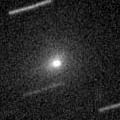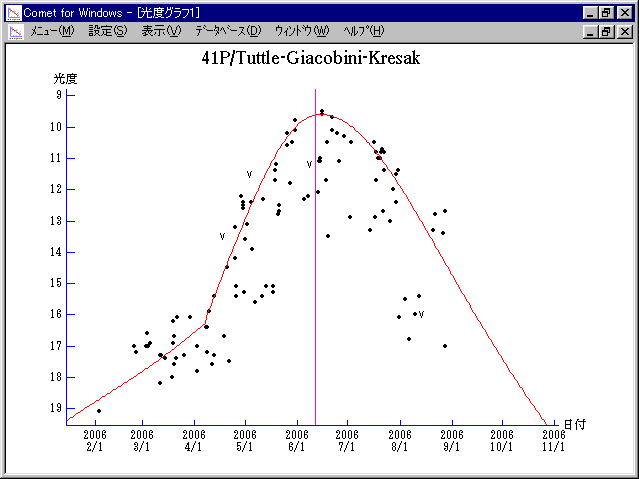# 41P/Tuttle-Giacobini-Kresak (2006)###Pictures###Orbital Elements

```Perturbed ephemeris below is based on elements from MPC 48383.

Epoch = 2453880.50000 TT   T = 2453897.77517 TT
Peri. =   62.19799         a =     3.0850459 AU
Node  =  141.08977 J2000.0 e =     0.6603720
Incl. =    9.22949         q =     1.0477681 AU
```

###Finding Charts###Magnitudes Graph

```        m1 = 13.5 + 5 log d + 18.0 log r  [   ,-65]  (             - 2006 Apr.  7)
m1 =  8.5 + 5 log d + 55   log r  [-65,  0]  (2006 Apr.  7 - 2006 June 11)
m1 =  9.0 + 5 log d + 30   log r  [  0,   ]  (2006 June 11 -             )
```##### The orbital elements are calculated using the Minor Planet & Comet Ephemeris Service. The charts are made with StellaNavigator Ver.2.0 for Windows (AstroArts / ASCII). The magnitudes graphs are made with Comet for Windows.